# How to Sum in Horizontal Range

To add numbers together we need to apply SUM function. But if we want to add numbers based on some conditions, we can add criteria with the help of SUMIFS function, SUMIFS can filter data with multiple criteria effectively. In this article, we will introduce you the method of applying SUMIFS function about sum numbers from different horizontal ranges.

We will introduce you the syntax, arguments of SUMIFS function, and let you know how this formula works to reach your goal. After reading the article, you may have a simple understanding of SUMIFS function and know how can we use this function to sum numbers with horizontal range.

## EXAMPLE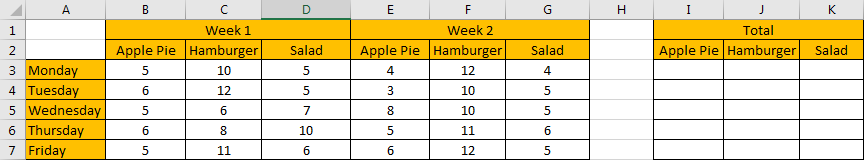Refer to the left-hand side table, it lists the amount for ‘Apple Pie’, ‘Hamburger’ and ‘Salad’ on weekdays (from ‘Monday’ to ‘Friday’) in two weeks. Start from row 3, in horizontal, it lists the amount for the three kinds of food on each weekday in two weeks. In the right-hand side table, it records the total amount for the three kinds of food in two weeks.

Now, we need to create a formula that can return the total amount for each food in two weeks based on different weekdays. This question can be seen as we need to sum data from a horizontal range with specific criteria. For example, in this instance, in I3, we want to enter a formula that can return the total amount for ‘Apple Pie’ on ‘Monday’ in ‘two weeks’ (week1 and week2). Besides, when dragging the formula to other cells (in range reference I3 to K7), we need the formula can adjust cell reference and range reference automatically and return the correct result based on updated parameters properly.

In this instance, we need to pick up numbers with criteria from each horizontal range, then sum them together. To fix this issue by formula, we can apply excel built-in function SUMIFS here.

## FORMULA – SUMIFS FUNCTIONS

Step 1: In I3, enter the formula =SUMIFS(\$A3:\$G3,\$A\$2:\$G\$2,I\$2).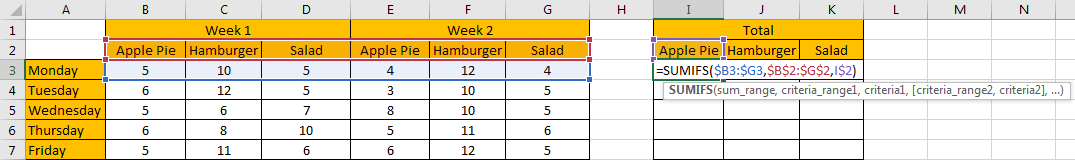Step 2: Press Enter after typing the formula.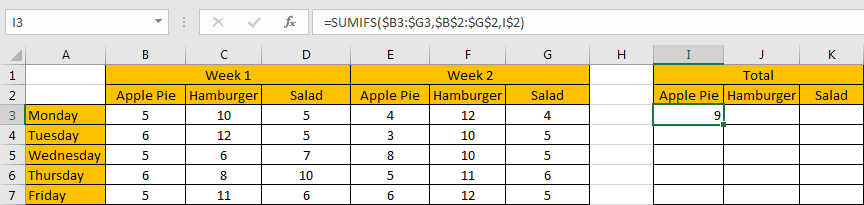We can see in cell I3 result 9 is returned, it is equal to 5 from B3 plus 4 from E3, the formula works correctly.

Step 3: Drag the ‘Auto Fill’ handle to make range reference I3 to K7 filled with the formula. Then we can see that results are automatically calculated and displayed in each cell correctly.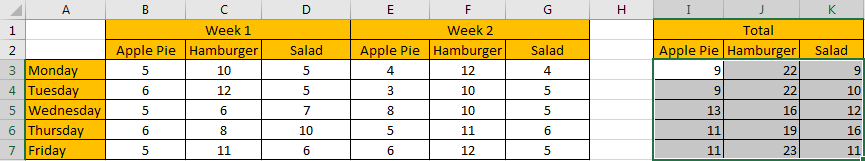## FUNCTION INTRODUCTION

SUMIFS function can be seen as SUM+IFS, it can handle multiple ‘criteria range’ and ‘criteria’ combinations.

Syntax:

`=SUMIFS(sum_range, criteria_range1, criteria1, [criteria_range2, criteria2], ...)`

[] part can be omitted.

For SUMIFS function, it supports wildcards like asterisk ‘*’ and question mark ‘?’, also support logical operators. If wildcards or logical operators are required, they should be enclosed into double quotes (““).

The usage of wildcards:

1. An asterisk (*) means one or more characters.
2. A question mark (?) means one character.
3. The position of asterisk or question mark means the character(s) position relative to the entered part.

The usage of logical operators:

1. “>” – greater than
2. “<” – less than
3. “<>” – not equal to

ALL ARGUMENTS

The formula is =SUMIFS(\$B3:\$G3,\$B\$2:\$G\$2,I\$2). Refer to above SUMIFS function introduction, we will split it to several parts.

SUMIFS – SUM RANGE

\$B3:\$G3 is the ‘sum range’ in this case.

You can see we add “\$” before column index A and G, that’s because when dragging the formula from I3 to J3 (formula is moved in horizontal direction), cell reference will be moved in horizontal by default, so we need add “\$” before columns to lock the range in horizontal direction, make sure the sum range can only be adjusted in vertical, but in horizontal, it is absolutely fixed.

See screenshot below, if “\$” is omitted, formula is updated to SUMIFS(C3:H3,\$B\$2:\$G\$2,J\$2) in J3, sum range is moved from range reference B3:G3 to C3:H3 improperly, the returned result is 9 incorrectly.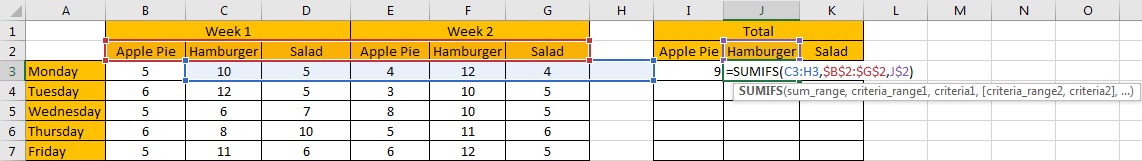Select \$B3:\$G3 from formula in the formula bar, the press F9, values in this range are expanded in an array.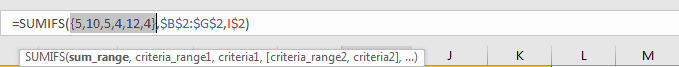### SUMIFS – CRITERIA RANGE

\$B\$2:\$G\$2 is the criteria range. We have only one criteria range in this instance.

Criteria range contains three kinds of food, due to there are two weeks, so we list them twice.

You can see we add “\$” before column and row index both, that’s because range \$B\$2:\$G\$2 is an absolute range. No matter copy formula to which cell reference, the criteria range is still \$B\$2:\$G\$2 and will not be changed. So we add ‘\$’ to lock this range.

Select \$B\$2:\$G\$2 from formula in the formula bar, the press F9, values in this range are expanded in an array.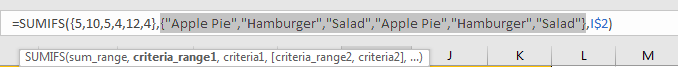### SUMIFS – CRITERIA

I\$2 is the criteria. I\$2=”Apple Pie”.

As we want to calculate total amount for the provided food, so can set criteria as I2 (I2=”Apple Pie”), J2 (J2=”Hamburger”), and K2 (K2=”Salad”). As row 2 is fixed, so we add ‘\$’ before row index 2.

We can also enter food name directly, as SUMIFS function supports texts, but they should be enclosed into double quotes “”, so for criteria “Apple Pie”, we can directly enter “Apple Pie” in criteria argument.

Select I\$2 from formula in the formula bar, the press F9, criteria value is displayed.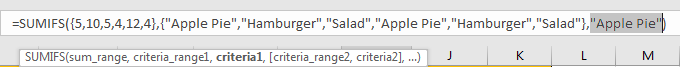## HOW THE FORMULA WORKS

After explaining each argument in the formula, now we will show you how the formula works with these arguments.

After expanding values in each range reference or cell reference, in the formula bar, the formula is displayed as:

`=SUMIFS({5,10,5,4,12,4},{"Apple Pie","Hamburger","Salad","Apple Pie","Hamburger","Salad"},"Apple Pie")`

There is one pair of criteria range and criteria.

{“Apple Pie”} – Criteria

If values from the criteria range are matched with the criteria “Apple Pie”, “True” will be recorded and saved, otherwise, “False” will be saved instead. So, after comparing, we can get a new array:

{True,False,False,True,False,False} – after comparing with criteria “Apple Pie”

For the following logical operation, “True” is coerced to ‘1’ and ‘False’ is coerced to ‘0’. So above array is converted to below array which consists of numbers “1” and “0”.

{1,0,0,1,0,0} – for criteria “Apple Pie”

Now, we have below two pairs of arrays:

{5,10,5,4,12,4} – sum range

{1,0,0,1,0,0} – for criteria “Apple Pie”

Elements are vertical-aligned in the two arrays. Multiply the two elements in the same position in the array. Then we can get a new array.

{5,0,0,4,0,0}

Add all products in above array, we get 9. Actually, in step#2, SUMIFS function returns 9 after calculation. For the other cells, the workflow is similar.

1. In this case, as we have only one pair of criteria range and criteria, we can also apply SUMIF function as well. The difference is SUMIF function only supports one group of criteria range and criteria, and its sum range is listed at the end.

Enter =SUMIF(\$B\$2:\$G\$2,I\$2,\$B3:\$G3), we can get the same result as applying SUMIFS.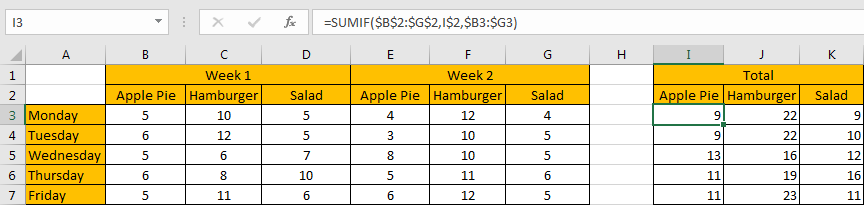### SUMMARY

1. SUMIFS function can handle multiple groups of criteria ranges and criteria. Sum range is the first argument among all arguments.

2. It supports user defined range name.

3. It supports wildcard.

4. It supports logical operators.

### Related Functions

• Excel SUMIF Function
The Excel SUMIF function sum the numbers in the range of cells that meet a single criteria that you specify. The syntax of the SUMIF function is as below:=SUMIF (range, criteria, [sum_range])…
• Excel SUMIFS Function
The Excel SUMIFS function sum the numbers in the range of cells that meet a single or multiple criteria that you specify. The syntax of the SUMIFS function is as below:=SUMIFS (sum_range, criteria_range1, criteria1, [criteria_range2, criteria2], …)…
• Excel SUM function
The Excel SUM function will adds all numbers in a range of cells and returns the sum of these values. You can add individual values, cell references or ranges in excel.The syntax of the SUM function is as below:= SUM(number1,[number2],…)
Related Posts

Average Of Numbers With Multiple Criteria In Excel

Have you ever come across a task to calculate the average of the numbers with respect to multiple criteria? Are you tired of doing this cumbersome task manually? Are you willing to do this task smartly in just a matter ...

How to Sum if Equal to Many Items or A Range in Excel

If we want to sum numbers from a range with criteria, we often select SUMIF of SUMIFS functions as the first choice to create a formula. The criteria can be a number or an array or a collection of some ...

How to Sum if Equal to X or Y in Excel

In daily work, if we want to sum numbers from a range, and only sum the numbers which being equal to X or Y in the range, we can create a formula with Excel build-in functions to get the result. ...

How to Sum Last N Days in Excel

In our daily life, we may want to sum amounts or sales for a specific period, for example in last N days. Sum numbers in Excel is easy to run, we can apply SUM function. But if we want to ...

How to Sum if Contains an Asterisk

To add numbers together we need to apply SUM function. And if we want to add numbers based on some conditions, we can add criteria with the help of SUMIFS function, SUMIFS can filter data with multiple criteria effectively. If ...

How to Sum in Vertical Range

If we want to add numbers based on some conditions in Excel worksheet, we can add criteria with the help of SUMIFS function, SUMIFS can filter data with multiple criteria effectively. In this article, we will introduce you the method ...

How to Sum with Criteria and Or Logic in Excel

To add numbers together we need to apply SUM function. And if we want to add numbers based on some conditions, we can add criteria with the help of SUMIFS function, SUMIFS can filter data with multiple criteria effectively. If ...

How to Subtotal Values for Groups and Only Keep One Subtotal for A Group in Column

Sometimes we may meet the case that subtotal values for different groups and only record a subtotal value for one group in a column, for other cells in this group, keep them blank. Today we will introduce you how can ...

How to Sum by Formula If Cells Are Not Blank in Criteria Range

Sometimes we may meet the cases that some blank cells exist in criteria range or sum range. In most situations we will ignore them, so we need to filter data by ‘not blank/not empty’. Today we will introduce you how ...

How to Sum by Formula if Cell Ends with in Excel

We have introduced the method of sum numbers based on criteria “sum if cell begins with” (you can refer to ‘How to Sum Data if Begins with in Excel’ on our website). In this article, we will show you the ...

Sidebar